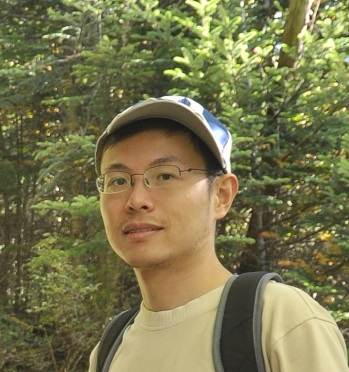# Molei Taoassociate professor (with tenure) School of Mathematics Georgia Institute of Technology Follow @MoleiTaoMath Office: Skiles 214A Address: 686 Cherry St NW, Atlanta GA 30332, USA Phone: +1-404-894-3711 mtao at gatech dot edu some other affiliations: GT Machine Learning Center Algorithms & Randomness Center (ARC) Algorithms, Combinatorics & Optimization (ACO) Program GT Decision & Control Lab

## What do I do?

As an applied & computational mathematician, I design and apply mathematical tools from nonlinear dynamics, probability, geometry, multiscale methods, numerical analysis and computational math, and control theory to solve practical problems.

An instance of applications is the mathematical foundation of machine learning, for which I'm interested in, for example,

• sampling, optimal transport, and (diffusion) generative modeling

• deep learning, and optimization

• scientific machine learning